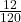## A train, initially moving at 12 m/s, speeds up to 36 m/s in 120 s. What is its acceleration?​

Question

A train, initially moving at 12 m/s, speeds up to
36 m/s in 120 s. What is its acceleration?​

in progress 0
5 months 2021-08-24T21:20:53+00:00 1 Answers 2 views 0

Acceleration is 35,9. I think so

Step-by-step explanation:

v0=12m/s

v=36m/s

t=120s

a=?

a=v–v0/t,

a=36 –a=36-0,1

a=35,9